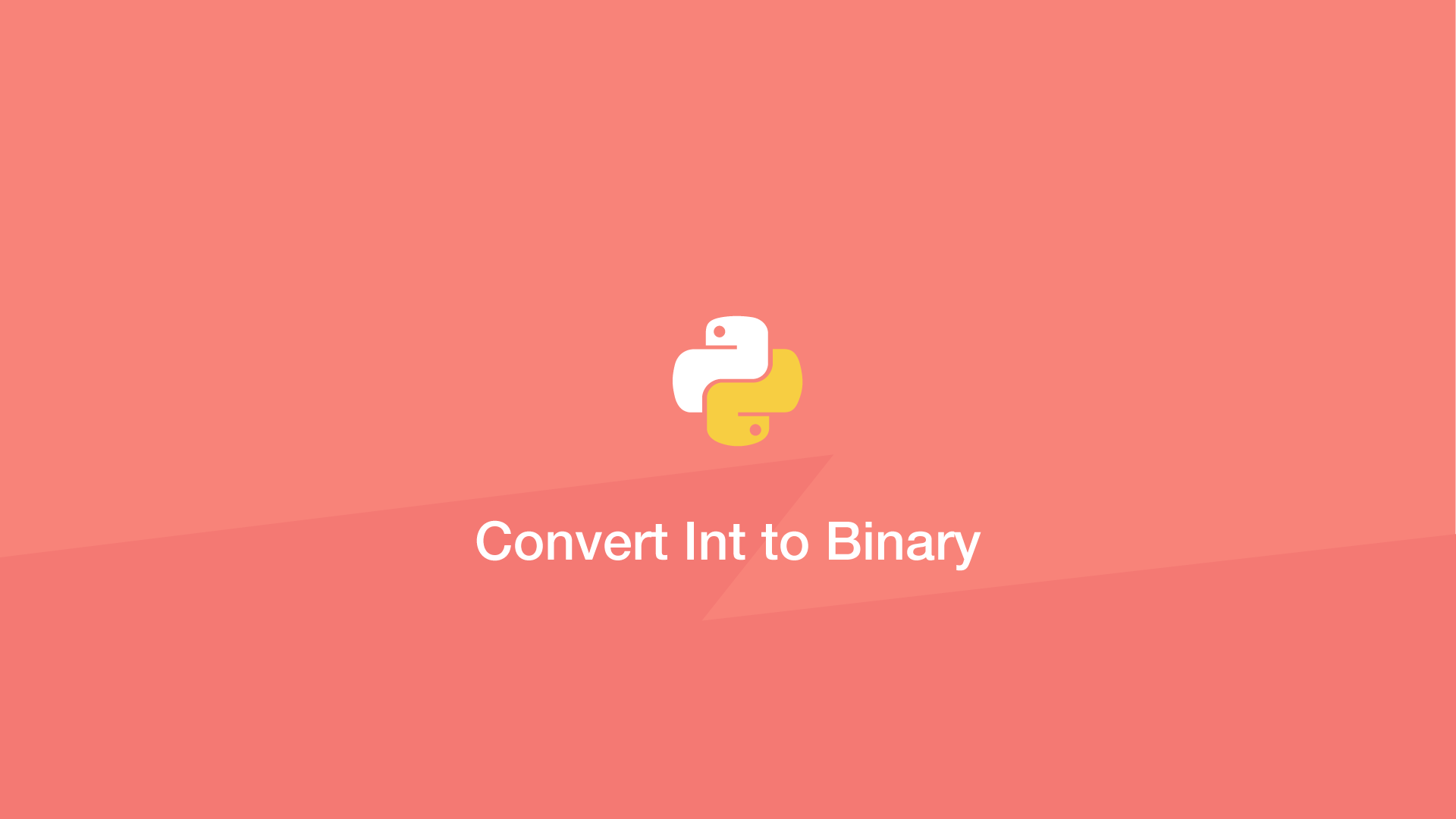# Convert Int to Binary in PythonTo convert an integer to binary in Python use either the bin() or format() function. In this tutorial, we will learn how to use both.

## Use bin() to Convert Int to Bin

In the example below, we will use the bin() function to change an int into binary representation.

``````num = 10

res = bin(num)

print(res)
``````
``````0b1010
``````

If you don't need the `0b` prefix, trim the first two characters like this:

``````num = 10

res = bin(num)[2:]

print(res)
``````
``````1010
``````

## Use format() to Convert Integer to Binary

The Python `format()` function can change integers to binary by setting the formatting type (the second argument) as `"b"`. Here is an example:

``````num = 10

res = format(num, "b")

print(res)
``````
``````1010
``````

#### Related Tutorials### How to Convert Hexadecimal to Binary in Python

November 22, 2021### How to Convert a String to a Number in Python

October 01, 2020### How to Add Two Hex Numbers in Python

November 16, 2021### How to Convert int to a char in Python

April 27, 2021### Python Type Conversion and Type Casting

June 14, 2021### Convert Hexadecimal to Decimal in Python

November 22, 2021